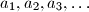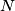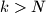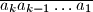### IMO Shortlist 2013 problem N4

Kvaliteta:
Avg: 0,0
Težina:
Avg: 7,0
Determine whether there exists an infinite sequence of nonzero digits$a_1, a_2, a_3, \ldots$ and a positive integer$N$ such that for every integer$k > N$, the number$\overline{a_k a_{k-1} \ldots a_1}$ is a perfect square.
Izvor: Iran